# Modulus of an annulus

(diff) ← Older revision | Latest revision (diff) | Newer revision → (diff)

The value reciprocal to the extremal length of the family of closed curves in the annuluswhich separate the boundary circles; the modulus is equal to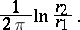By a conformal mapping onto an associated annulus, the modulus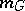of an annular domaincan be obtained. It turns out that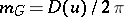, where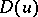is the Dirichlet integral of the real part of the functionmappingonto: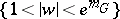. (Thus, a given annular domain is mapped onto an annulus with a fixed ratio of the radii of the boundary circles. This fact can be taken as another definition of the modulus of an annulus, its generalization leads to the idea of the modulus of a plane domain.)

A generalization of the modulus of an annular domain is the modulus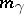of a prime end (cf. Cluster set; Limit elements)of an open Riemann surfacerelative to a neighbourhood. Depending on whetheris finite or infinite, the prime end has hyperbolic or parabolic type andeither does or does not have a Green function.

For a simply-connected domainof hyperbolic type the so-called reduced modulus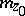relative to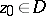is defined as the limit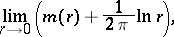whereis the modulus of the annular domain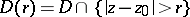. It turns out that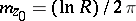, whereis the conformal radius (cf. Conformal radius of a domain) ofrelative to.